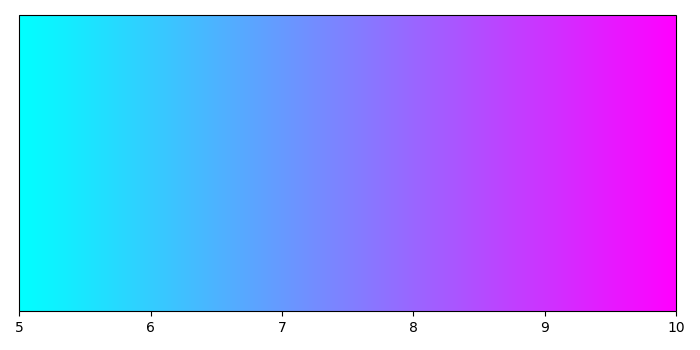# How to build colorbars without attached plot in matplotlib?

To build colorbars without attached plot in matplotlib, we can take the following steps.

## Steps

• Set the figure size and adjust the padding between and around the subplots.

• Create a figure and a set of subplots.

• Adjust the subplot layout parameters.

• Normalize the quaternion in place. Return the norm of the quaternion.

• Get the colorbar instance (cb) with base colorbar and horizontal orientation.

• To display the figure, use Show() method.

## Example

import matplotlib.pyplot as plt
import matplotlib as mpl

# Set the figure size
plt.rcParams["figure.figsize"] = [7.00, 3.50]
plt.rcParams["figure.autolayout"] = True

# Create a figure and a set of subplots
fig, ax = plt.subplots()

# Adjust the subplot layout parameter

# Normalize
norm = mpl.colors.Normalize(vmin=5, vmax=10)

# Get the colorbar instance
cb = mpl.colorbar.ColorbarBase(ax, cmap=mpl.cm.cool, norm=norm, orientation='horizontal')

# Display the plot
plt.show()

## Output

It will produce the following output −